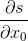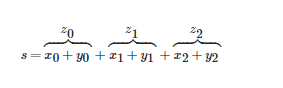# PyTorch简介

## 1.Torch张量库介绍

``````# 作者: Robert Guthrie

import torch
import torch.nn as nn
import torch.nn.functional as F
import torch.optim as optim

torch.manual_seed(1)
``````

#### 1.1 创建张量

``````# 利用给定数据创建一个torch.Tensor对象.这是一个一维向量
V_data = [1., 2., 3.]
V = torch.Tensor(V_data)
print(V)

# 创建一个矩阵
M_data = [[1., 2., 3.], [4., 5., 6]]
M = torch.Tensor(M_data)
print(M)

# 创建2x2x2形式的三维张量.
T_data = [[[1., 2.], [3., 4.]],
[[5., 6.], [7., 8.]]]
T = torch.Tensor(T_data)
print(T)
``````
• 输出结果：
``````tensor([1., 2., 3.])
tensor([[1., 2., 3.],
[4., 5., 6.]])
tensor([[[1., 2.],
[3., 4.]],

[[5., 6.],
[7., 8.]]])
``````

``````# 索引V得到一个标量（0维张量）
print(V)

# 从向量V中获取一个数字
print(V.item())

# 索引M得到一个向量
print(M)

# 索引T得到一个矩阵
print(T)
``````
• 输出结果：
``````tensor(1.)
1.0
tensor([1., 2., 3.])
tensor([[1., 2.],
[3., 4.]])
``````

``````x = torch.randn((3, 4, 5))
print(x)
``````
• 输出结果：
``````tensor([[[-1.5256, -0.7502, -0.6540, -1.6095, -0.1002],
[-0.6092, -0.9798, -1.6091, -0.7121,  0.3037],
[-0.7773, -0.2515, -0.2223,  1.6871,  0.2284],
[ 0.4676, -0.6970, -1.1608,  0.6995,  0.1991]],

[[ 0.8657,  0.2444, -0.6629,  0.8073,  1.1017],
[-0.1759, -2.2456, -1.4465,  0.0612, -0.6177],
[-0.7981, -0.1316,  1.8793, -0.0721,  0.1578],
[-0.7735,  0.1991,  0.0457,  0.1530, -0.4757]],

[[-0.1110,  0.2927, -0.1578, -0.0288,  0.4533],
[ 1.1422,  0.2486, -1.7754, -0.0255, -1.0233],
[-0.5962, -1.0055,  0.4285,  1.4761, -1.7869],
[ 1.6103, -0.7040, -0.1853, -0.9962, -0.8313]]])
``````

#### 1.2 张量操作

``````x = torch.Tensor([1., 2., 3.])
y = torch.Tensor([4., 5., 6.])
z = x + y
print(z)
``````
• 输出结果：
``````tensor([5., 7., 9.])
``````

``````# 默认情况下, 它沿着第一个行进行连接 (连接行)
x_1 = torch.randn(2, 5)
y_1 = torch.randn(3, 5)
z_1 = torch.cat([x_1, y_1])
print(z_1)

# 连接列：
x_2 = torch.randn(2, 3)
y_2 = torch.randn(2, 5)
# 第二个参数指定了沿着哪条轴连接
z_2 = torch.cat([x_2, y_2], 1)
print(z_2)

# 如果你的tensors是不兼容的，torch会报错。取消注释来查看错误。
# torch.cat([x_1, x_2])
``````
• 输出结果：
``````tensor([[-0.8029,  0.2366,  0.2857,  0.6898, -0.6331],
[ 0.8795, -0.6842,  0.4533,  0.2912, -0.8317],
[-0.5525,  0.6355, -0.3968, -0.6571, -1.6428],
[ 0.9803, -0.0421, -0.8206,  0.3133, -1.1352],
[ 0.3773, -0.2824, -2.5667, -1.4303,  0.5009]])
tensor([[ 0.5438, -0.4057,  1.1341, -0.1473,  0.6272,  1.0935,  0.0939,  1.2381],
[-1.1115,  0.3501, -0.7703, -1.3459,  0.5119, -0.6933, -0.1668, -0.9999]])
``````

#### 1.3 重构张量

``````x = torch.randn(2, 3, 4)
print(x)
print(x.view(2, 12))  # 重构为2行12列
# 同上。如果维度为-1,那么它的大小可以根据数据推断出来
print(x.view(2, -1))
``````
• 输出结果：
``````tensor([[[ 0.4175, -0.2127, -0.8400, -0.4200],
[-0.6240, -0.9773,  0.8748,  0.9873],
[-0.0594, -2.4919,  0.2423,  0.2883]],

[[-0.1095,  0.3126,  1.5038,  0.5038],
[ 0.6223, -0.4481, -0.2856,  0.3880],
[-1.1435, -0.6512, -0.1032,  0.6937]]])
tensor([[ 0.4175, -0.2127, -0.8400, -0.4200, -0.6240, -0.9773,  0.8748,  0.9873,
-0.0594, -2.4919,  0.2423,  0.2883],
[-0.1095,  0.3126,  1.5038,  0.5038,  0.6223, -0.4481, -0.2856,  0.3880,
-1.1435, -0.6512, -0.1032,  0.6937]])
tensor([[ 0.4175, -0.2127, -0.8400, -0.4200, -0.6240, -0.9773,  0.8748,  0.9873,
-0.0594, -2.4919,  0.2423,  0.2883],
[-0.1095,  0.3126,  1.5038,  0.5038,  0.6223, -0.4481, -0.2856,  0.3880,
-1.1435, -0.6512, -0.1032,  0.6937]])
``````

## 2.计算图和自动求导

``````# 张量对象带有“requires_grad”标记

y = torch.Tensor([4., 5., 6], requires_grad=True)
z = x + y
print(z)

# 但是z还有一些额外的东西.
``````
• 输出结果：
``````tensor([5., 7., 9.], grad_fn=<AddBackward0>)
``````

``````# 我们来将z中所有项作和运算
s = z.sum()
print(s)
``````
• 输出结果：
``````tensor(21., grad_fn=<SumBackward0>)
<SumBackward0 object at 0x7fd73f1c7e48>
``````s是被作为张量z的和创建的。张量z是x+y的和，因此``````# 在任意变量上使用 .backward()将会运行反向,从它开始.
s.backward()
``````
• 输出结果;
``````tensor([1., 1., 1.])
``````

``````x = torch.randn((2, 2))
y = torch.randn((2, 2))
z = x + y
# 你不能通过z反向传播。

# 如果没有指定，标记默认为True
#正如我们在上面看到的一样，z包含足够的信息计算梯度。
z = x + y

# 现在z有关于x,y的历史信息
# 我们可以获取它的值，将其从历史中分离出来吗？
new_z = z.detach()

# new_z有足够的信息反向传播至x和y吗？
# 答案是没有
# 怎么会这样？ “z.detach()”函数返回了一个与“z”相同存储的张量
#但是没有携带历史的计算信息。
# 它对于自己是如何计算得来的不知道任何事情。
# 从本质上讲，我们已经把这个变量从过去的历史中分离出来了。
``````
• 输出结果：
``````False False
None
True
None
``````

``````print(x.requires_grad)
``````True